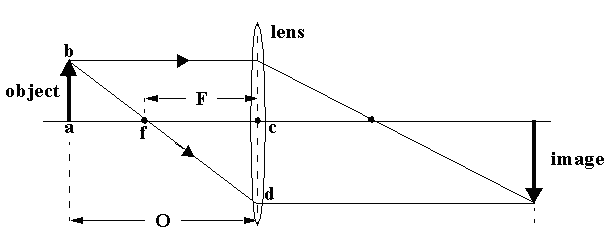# Effective Focal Length of a Lens and Plane Port

What is the effective focal length of a lens used underwater behind a plane port? A thin bi-convex lens of focal length F in air produces an inverted real image as shown in the figure. The ratio of image height to object height, cd/ab, is the called the magnification 'm'. From the similar triangles abf and cdf this ratio is also fc/fa, or

m = F/(O - F)F'/(O - F') = F/(0.75*O - F)

F' = 1.33*F